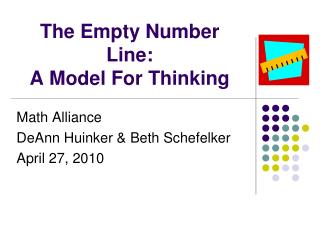DownloadDownload PresentationThe Empty Number Line:

# The Empty Number Line:

Télécharger la présentation## The Empty Number Line:

- - - - - - - - - - - - - - - - - - - - - - - - - - - E N D - - - - - - - - - - - - - - - - - - - - - - - - - - -
##### Presentation Transcript

1. The Empty Number Line: A Model For Thinking Math Alliance DeAnn Huinker & Beth Schefelker April 27, 2010

2. Representations:Models for Thinking Strengthening the ability to move between and among representations improves the growth of children’s conceptual understanding. Vandewalle, J. Elementary and Middle School Mathematics Teaching Developmentally. Pearson Education, 2007. . pictures manipulative models oral language written symbols Real-world situations

3. Models for thinking A model for thinking about a mathematical concept refers to any object, picture, or drawing that represents the concept. • To see a concept in a model you must have some relationship in your mind to impose on the model. • Models give children something to think about, explore with, talk about, and reason with.

4. What is Cosette thinking? The book cart has an assortment of things to read for indoor recess. Out of the 70 books, 37 are comic books. How many are not comic books? Solve it (no standard algorithms allowed). Then turn & share.

5. Cosette’s Model • What mathematical understanding is Cosette demonstrating in her thinking? • How is she thinking about 70 – 37 = ? • How would she solve 80 – 52 = ?

6. The empty number line: A useful tool or just another procedure?Bobis, J. Teaching Children Mathematics. April 2007 • What are the benefits for using an open number line for students? • What are the benefits for using an open number line for teachers? • What needs to be considered when introducing the open number line to students?

7. A. Counting by 10 23 + 40 67 + 20 B. Bridging to 10 7 + 8 38 + 5 C. Jump Strategy 46 – 39 72 – 51 D. Compensation Strategy 45 – 29 57 + 39 Try the strategies in the article Each person selects one strategy. Practice it. Use an empty number line, if appropriate. Then teach it to your table partners. E. Split Strategy • 35 – 21 • 56 + 32 What connections are you finding to the strategies we have explored and discussed in class?

8. Looking At Student Work • Pass out the four pieces of student work. • Analyze what the student is doing. • How are students keeping track of their thinking? • What relationships are students demonstrating as they use the empty number line as a model for their thinking? • How is the work similar? How is it different? • Can you connect the work to any of the strategies from the article?

9. The book cart has an assortment of things to read forindoor recess. Out of the 70, books 37 are comic books. How many are not comic books? How would you explain why you “add” the 1?

10. Big ideas behind the Empty Number Line • Numbers can be decomposed and the subunits or smaller amounts can be added or subtracted in varying orders, yet still be equivalent. • 25 +17 = 25 + 10 + 7 25 + 10 + 5 + 2 25 + 5 + 10 + 2 • Place value patterns occur when adding on groups of ten. • 27, 37, 47, 57 • Unitizing • 1 jump of 10 is the same as counting 10 units

11. Study of Sam How does Sam use his understanding of number and relationships as he works on the math problems? • 3rd Grader at Elm Creative Arts

12. 26 + 39 = ____ + 27clip starts at 2:10 • Solve the problem. • What mathematical understandings are evidenced in this work? SAM • What does Sam know about the math? • What is Sam struggling with as he works? • What instructional moves were made by the teacher?

13. 71 – 69 = ?clip starts at 5:29 • Solve the problem. • Share your thinking with a neighbor. SAM • What does Sam know about the math? • What is Sam struggling with as he works? • What instructional moves were made by the teacher?

14. 14 – 6 = ? • Turn and talk • Describe Sam’s method. SAM • What does Sam know about the math? • What instructional moves were made by the teacher?

15. Meet Grant30:13 How does Grant approach solving… 25 + 37 How did the model help Grant explain his thinking?

16. What are you walking away with. . . ? • What thoughts are you walking away with as you thought about the instructional moves in the video clips? • In what ways do models for thinking support the development of computational fluency?